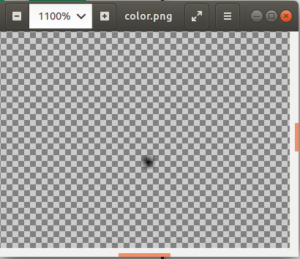Open in App
Not now

# Wand alpha() function in Python

• Last Updated : 16 Mar, 2023

The alpha() function is identical to the wand color() function. Similar to color() function, alpha() function draws a color on the image using current fill color, starting at specified position & method. Uses same arguments as color() method.

Syntax: wand.drawing.alpha(x, y, method) Parameters :

The following are the PAINT_METHOD_TYPES:

• ‘point’ alters a single pixel.
• ‘replace’ swaps on color for another. Threshold is influenced by fuzz.
• ‘floodfill’ fills area of a color influenced by fuzz.
• ‘filltoborder’ fills area of a color until border defined by border_color.
• ‘reset’ replaces the whole image to a single color.

Note: This method replaces matte() in ImageMagick version 7. An AttributeError will be raised if attempting to call on a library without DrawAlpha support. Example #1:

## Python3

 `# Import required objects from wand modules``from` `wand.image ``import` `Image``from` `wand.drawing ``import` `Drawing``from` `wand.color ``import` `Color` `# generate object for wand.drawing``with Drawing() as draw:``    ``draw.alpha(``100``, ``100``, ``'point'``)``    ``with Image(width ``=` `200``,``               ``height ``=` `200``) as img:` `        ``# draw shape on image using draw() function``        ``draw.draw(img)``        ``img.save(filename ``=``'color.png'``)`

Output: A pixel is visible at center of transparent image here is the zoomed image.Example #2: Filling color using a flood-fill algorithm.

## Python3

 `# Import required objects from wand modules``from` `wand.image ``import` `Image``from` `wand.drawing ``import` `Drawing``from` `wand.color ``import` `Color` `# generate object for wand.drawing``with Drawing() as draw:``    ``draw.fill_color ``=` `Color(``'blue'``)``    ``draw.alpha(``10``, ``35``, ``'floodfill'``)``    ``with Image(width ``=` `200``,``               ``height ``=` `200``,``               ``background ``=` `Color(``'white'``)) as img:` `        ``# draw shape on image using draw() function``        ``draw.draw(img)``        ``img.save(filename ``=``'color2.png'``)`

Output:My Personal Notes arrow_drop_up